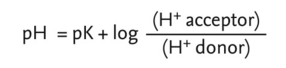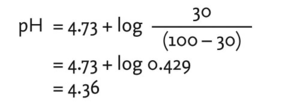# Henderson-Hasselbach equation

## Henderson-Hasselbach equation

the formula for calculating the pH of a buffer solution, which states that:where pK is the dissociation constant of an acid. The variable term is really a ratio of concentration values and can be taken as being proportional to the amount of acid and base added in the buffer. For example, when 30 cm3 of 0.1 M NaOH is added to 100 cm3 of 0.1 M acetic acid and the pK for acetic acid is 4.73, we can estimate:Collins Dictionary of Biology, 3rd ed. © W. G. Hale, V. A. Saunders, J. P. Margham 2005
References in periodicals archive ?
The theoretical OF/P ratio should be around 3.1 as calculated using the Henderson-Hasselbach equation (19).
For a weak acid, the pH at any time can be described by the Henderson-Hasselbach equation:
Effect of the variation of pK of the Henderson-Hasselbach equation on values obtained for total C[O.sub.2], calculated from pC[O.sub.2] and pH values.
A modified version of the Henderson-Hasselbach equation, which uses the [pK.sub.a] and the pH, allows theoretical calculation of the fluid-to-plasma concentration ratio (F/P ratio) (3).
Site: Follow: Share:
Open / Close